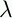September 22, 1999 Perl and the Lambda Calculus Slide #24

# Ordered Pair Example

(SECOND (PAIR M N))

(p.(p FALSE) (PAIR M N))

((PAIR M N) FALSE)

((xy.b.(b x y) M N) FALSE)

((y.b.(b M y) N) FALSE)

(b.(b M N) FALSE)

(FALSE M N)

(pq.q M N)

(q.q N)

N

Which is what we had hoped for

A similar computation of (FIRST P) yields M.

 NextCopyright © 1999 M-J. Dominus